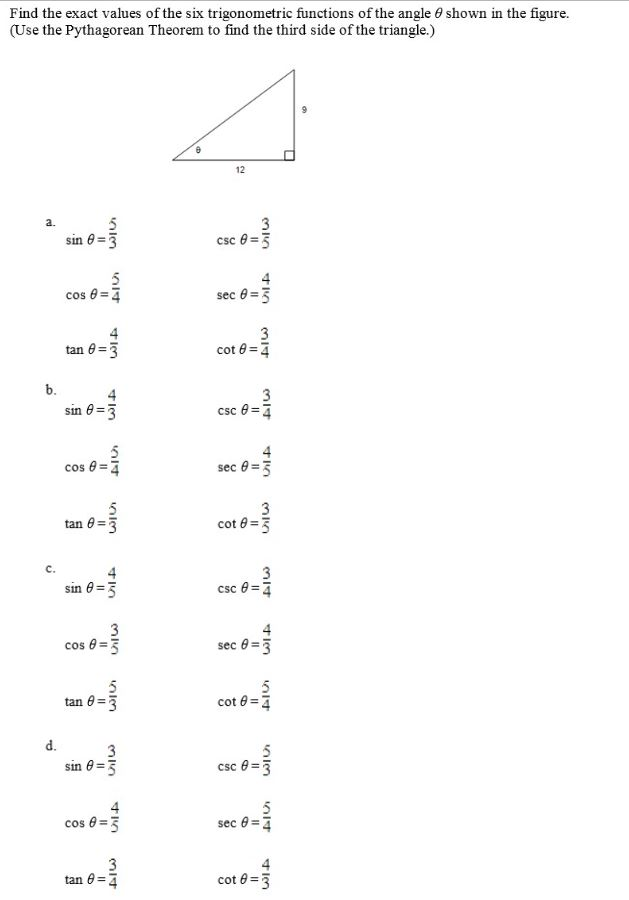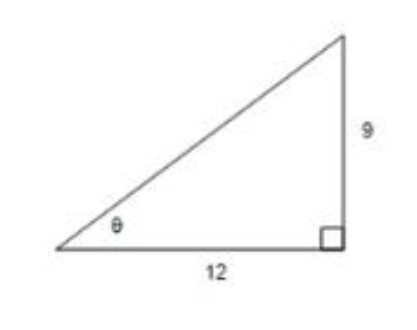# Find the exact values of the six trigonometric functions of the angle 6 shown in the figure.(Use the Pythagorean Theorem to find the third side of the triangle.)123a.sin 0 =3csc e =3sec 0=3cos 8 =3cot 0 =7tan 0 =3b.3csc e=4sin 0=3cos 8 =7sec e =33cot 8 =3tan 0 =3csc e=7sin e =34sec 8 =3cos 8tan 0 =3cot 0 =7d.csc e =3sin 0=3cos e =3sec @ =3tan 0 =7cot 8 =3II

Question
6 views

I am needing help with the equation in the attached image.help_outlineImage TranscriptioncloseFind the exact values of the six trigonometric functions of the angle 6 shown in the figure. (Use the Pythagorean Theorem to find the third side of the triangle.) 12 3 a. sin 0 =3 csc e =3 sec 0=3 cos 8 =3 cot 0 =7 tan 0 =3 b. 3 csc e=4 sin 0=3 cos 8 =7 sec e =3 3 cot 8 =3 tan 0 =3 csc e=7 sin e =3 4 sec 8 =3 cos 8 tan 0 =3 cot 0 =7 d. csc e =3 sin 0=3 cos e =3 sec @ = 3 tan 0 =7 cot 8 =3 II fullscreen
check_circle

Step 1

To determine the all the six trigonometric ratios.

Step 2

Given:Step 3

In the given right angled triangle,

h2 = 122+92

h2 = 144+81

h2 =225

### Want to see the full answer?

See Solution

#### Want to see this answer and more?

Solutions are written by subject experts who are available 24/7. Questions are typically answered within 1 hour.*

See Solution
*Response times may vary by subject and question.
Tagged in